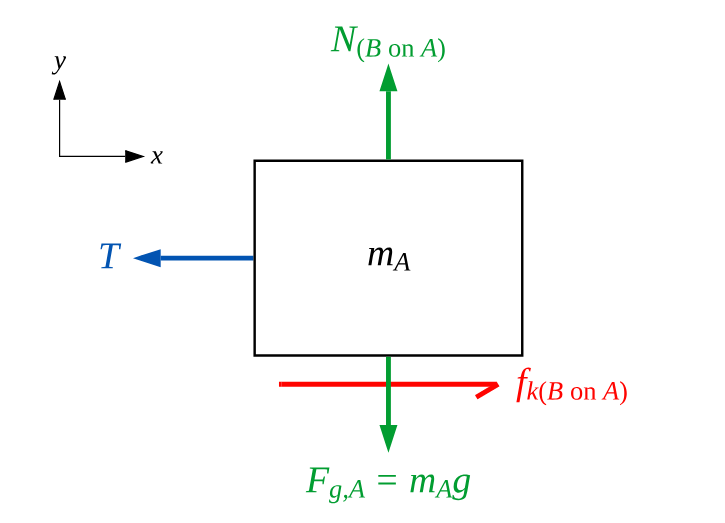# Problem: The 1.0 kg block in the figure below is tied to the wall with a rope. It sits on top of the 2.0 kg block. The lower block is pulled to the right with a tension force of 40 N. The coefficient of kinetic friction at both the lower and upper surfaces of the 2.0 kg block is μk = 0.35.What is the tension in the rope holding the 1.0 kg block to the wall?_____N

###### FREE Expert Solution

Friction force:

$\overline{){\mathbf{f}}{\mathbf{=}}{\mathbf{\mu }}{\mathbf{N}}}$

The normal force on the 1-kg top block, N = mg = (1)(9.8) = 9.8 N

FBD for the top block, which we'll call A:82% (101 ratings)###### Problem Details

The 1.0 kg block in the figure below is tied to the wall with a rope. It sits on top of the 2.0 kg block. The lower block is pulled to the right with a tension force of 40 N. The coefficient of kinetic friction at both the lower and upper surfaces of the 2.0 kg block is μk = 0.35.

What is the tension in the rope holding the 1.0 kg block to the wall?
_____N